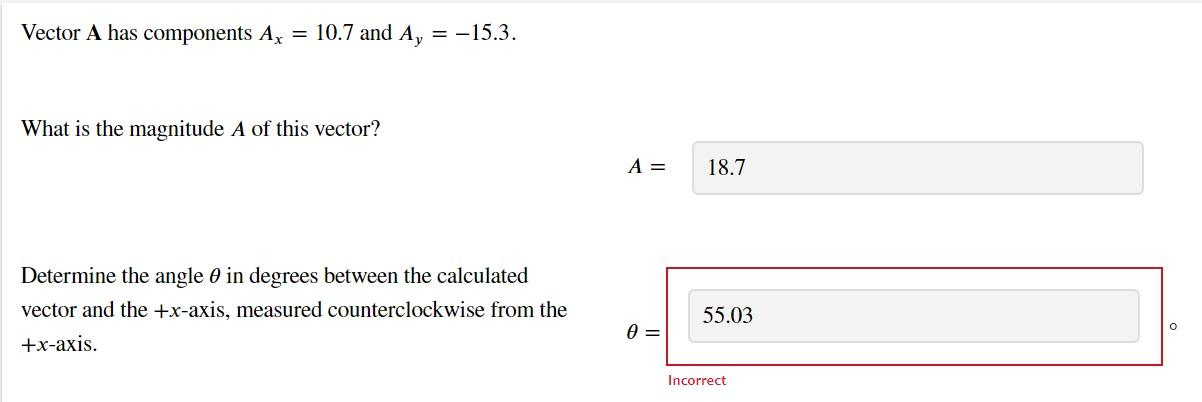Home / Expert Answers / Physics / vector-mathbf-a-has-components-a-x-10-7-and-a-y-15-3-what-is-the-magnitu-pa771

# (Solved): Vector $$\mathbf{A}$$ has components $$A_{x}=10.7$$ and $$A_{y}=-15.3$$. What is the magnitu ...Vector $$\mathbf{A}$$ has components $$A_{x}=10.7$$ and $$A_{y}=-15.3$$. What is the magnitude $$A$$ of this vector? $A=$ Determine the angle $$\theta$$ in degrees between the calculated vector and the $$+x$$-axis, measured counterclockwise from the $$+x$$-axis. $\theta=$ Incorrect

We have an Answer from Expert